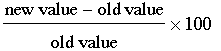SEARCH HOMEMath Central Quandaries & QueriesQuestion from Rebecca: In 2008 my land was valued at $84,000. In 2009 my land was re-valued at$235,000.00. What is the percent increase between the two values? I want to be able to express "the value was increased by x%."Rebecca,

Percentage change is calculated as the new value minus the old value, divided by the old value and then multiplied by 100. That isIn your case new value minus old value is $235,000 -$84,000 = $151,000 so the increase in value is$151,000. Divided by the old value gives $151,000/$84,000 = 1.80. Finally, multiplying by 100 yields 180%.

HarleyMath Central is supported by the University of Regina and The Pacific Institute for the Mathematical Sciences.